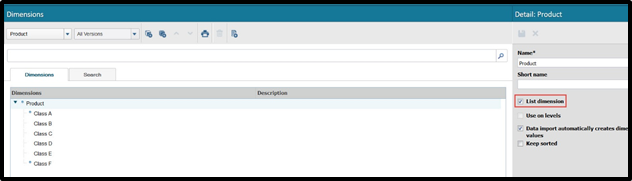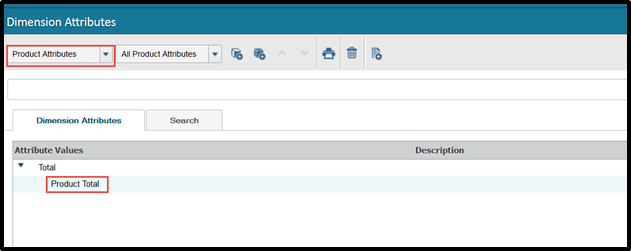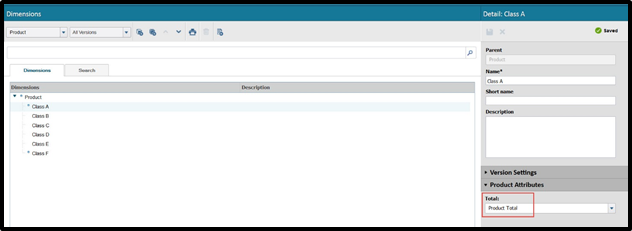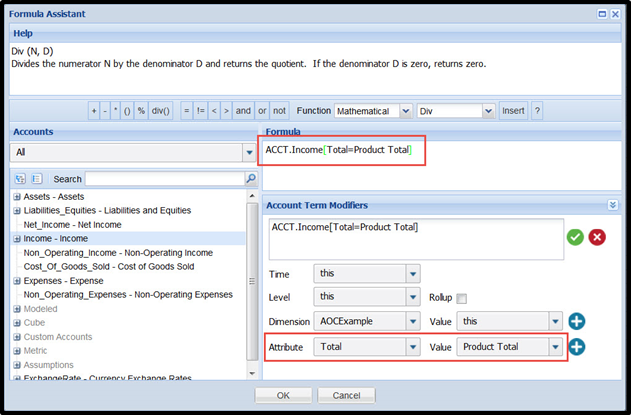メインコンテンツまでスキップ

# How Can I Reference The Total For A List Dimension?

## Question

How can I reference the total for a list dimension?

You can reference the total for a list dimension by tagging all list dimension values with a single dimension attribute and then referencing said attribute value in a formula. A more detailed example is below.

1) Create a new List dimension (let’s call this Product) from Modeling > Dimensions and add its associated values.Any new dimension will be a List dimension by default.

• Any new dimension will be a list dimension by default.
• A list dimension is a dimension without a hierarchy in its values.
• You could use an existing list dimension as well.2) From Modeling > Dimension Attributes, under Product Attributes,create a new dimension attribute (let’s call this Total) and a dimension attribute value (let’s call this Product Total).3) Going back to Modeling > Dimensions, tag all existing Product values with the dimension attribute value Product Total.4) You can now reference the Product Total dimension attribute value to represent a total for all Product values.• この記事は役に立ちましたか？